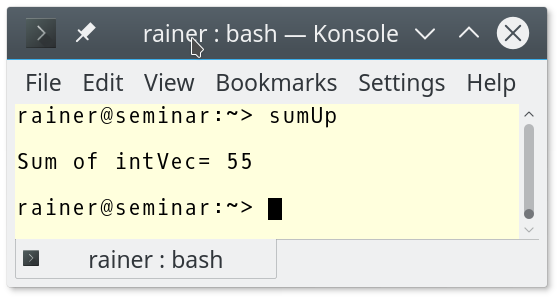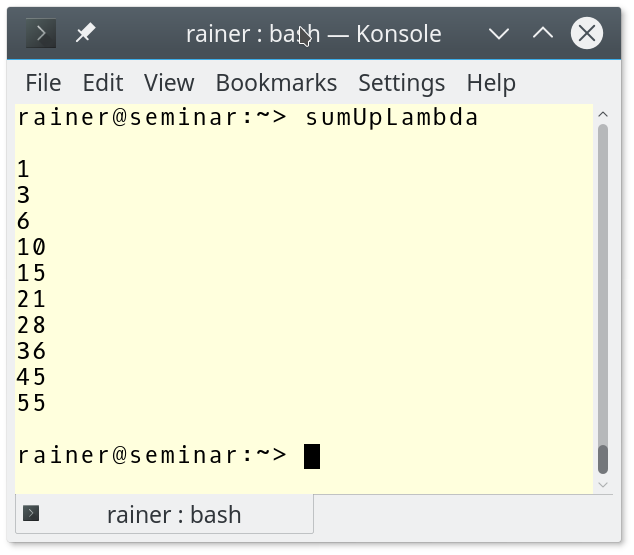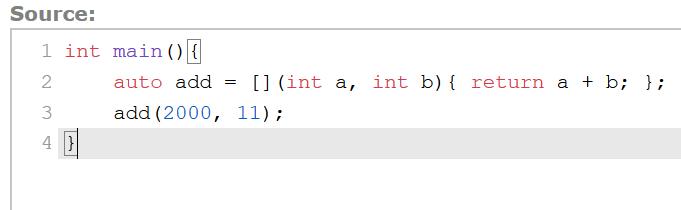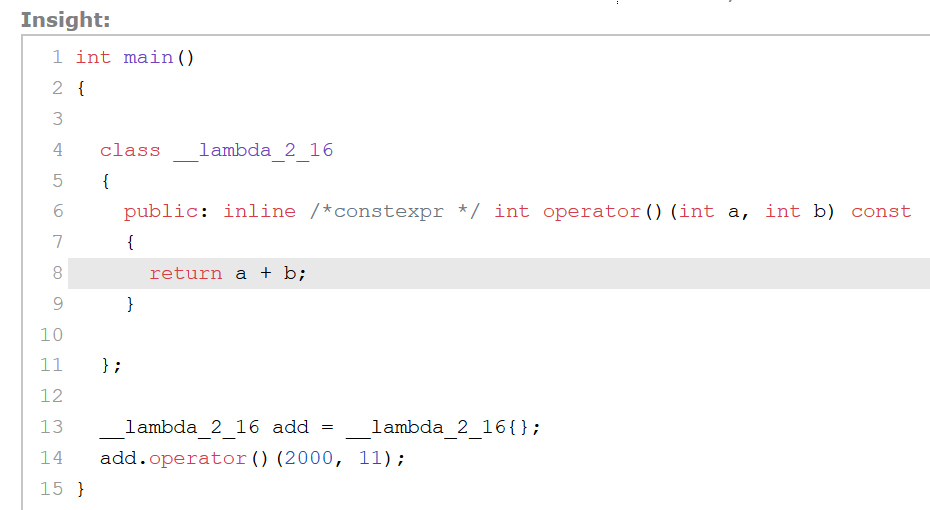# C++ Core Guidelines: Pass Function Objects as Operations

An interface is a contract between a user and an implementer and should, therefore, be written with great care. This also holds true if you pass an operation as an argument.Today, I’m just writing about rule 40 because function objects are used quite heavily in modern C++.

## T.40: Use function objects to pass operations to algorithms

First, you may be irritated that the rules didn’t explicitly mention lambda functions but use them. Later, I will write about this point in detail.

There are various ways to sort a vector of strings.

##Modernes C++ Mentoring

Be part of my mentoring programs:

• "Fundamentals for C++ Professionals" (open)
• "Design Patterns and Architectural Patterns with C++" (open)
• "C++20: Get the Details" (reopens December 2023)
• Do you want to stay informed about my mentoring programs: Subscribe via E-Mail.

```// functionObjects.cpp

#include <algorithm>
#include <functional>
#include <iostream>
#include <iterator>
#include <string>
#include <vector>

bool lessLength(const std::string& f, const std::string& s){      // (6)
return f.length() < s.length();
}

class GreaterLength{                                              // (7)
public:
bool operator()(const std::string& f, const std::string& s) const{
return f.length() > s.length();
}
};

int main(){

std::vector<std::string> myStrVec = {"523345", "4336893456", "7234",
"564", "199", "433", "2435345"};

std::cout << "\n";
std::cout << "ascending with function object" << std::endl;
std::sort(myStrVec.begin(), myStrVec.end(), std::less<std::string>()); // (1)
for (const auto& str: myStrVec) std::cout << str << " ";
std::cout << "\n\n";

std::cout << "descending with function object" << std::endl;
std::sort(myStrVec.begin(), myStrVec.end(), std::greater<>());         // (2)
for (const auto& str: myStrVec) std::cout << str << " ";
std::cout << "\n\n";

std::cout << "ascending by length with function" << std::endl;
std::sort(myStrVec.begin(), myStrVec.end(), lessLength);               // (3)
for (const auto& str: myStrVec) std::cout << str << " ";
std::cout << "\n\n";

std::cout << "descending by length with function object" << std::endl;
std::sort(myStrVec.begin(), myStrVec.end(), GreaterLength());          // (4)
for (const auto& str: myStrVec) std::cout << str << " ";
std::cout << "\n\n";

std::cout << "ascending by length with lambda function" << std::endl;
std::sort(myStrVec.begin(), myStrVec.end(),                           // (5)
[](const std::string& f, const std::string& s){
return f.length() < s.length();
});
for (const auto& str: myStrVec) std::cout << str << " ";
std::cout << "\n\n";

}
```

The program sorts a vector of strings lexicographically and based on the length of the strings. I used in lines (1) and (2) two function objects from the Standard template library. A function object is an instance of a class for which the call operator (operater ()) is overloaded. Often, there are falsely called functors. I hope, you notice the difference between the call std::sort(myStrVec.begin(), myStrVec.end(), std::less<std::string>()) in line (1) and std::sort(myStrVec.begin(), myStrVec.end(), std::greater<>())in line (2). The second expression (std::greater<>()), in which I provided no type for the predicate, has been valid since C++14. I sorted in lines (3), (4), and (5) by using a function (6), a function object (7), and a lambda function (5). This time, the length of the strings was the sorting criterion.

For completeness, here is the output of the program.The rules state you should

My argumentation includes three points: Performance, Expressiveness, and State. It makes my answer that lambda functions are function objects under the hood relatively easy.

#### Performance

The more the optimizer can reason locally, the more optimization is possible. A function object (4) or a lambda function (5) can be generated just in place. Compare this to a function that was defined in a different translation unit. If you don’t believe me, use the compiler explorer and compare the assembler instructions. Of course, compile with maximum optimization.

#### Expressiveness

“Explicit is better than implicit”. This meta-rule from Python also applies to C++. It means that your code should explicitly express its intent. Of course, this holds particularly for lambda functions such as line (5). Compare this with the function lessLength in line (6) used in line (3). Imagine your coworker would name the function foo; therefore, you have no idea what the function should do. You have to document its usage, such as in the following line.

```// sorts the vector ascending, based on the length of its strings
std::sort(myStrVec.begin(), myStrVec.end(), foo);
```

Further, you have to hope that your coworker wrote a correct predicate. If you don’t believe him, you must consider the implementation. Maybe that’s not possible because you have the declaration of the function. With a lambda function, your coworker can not fool you. The code is the truth. Let me put it more provocatively: Your code should be such expressive that it needs no documentation.

#### State

In contrast to a function, a function object can have a state. The code example makes my point.

```// sumUp.cpp

#include <algorithm>
#include <iostream>
#include <vector>

class SumMe{
int sum{0};
public:
SumMe() = default;

void operator()(int x){
sum += x;
}

int getSum(){
return sum;
}
};

int main(){

std::vector<int> intVec{1, 2, 3, 4, 5, 6, 7, 8, 9, 10};

SumMe sumMe= std::for_each(intVec.begin(), intVec.end(), SumMe());  // (1)

std::cout << "\n";
std::cout << "Sum of intVec= " << sumMe.getSum() << std::endl;      // (2)
std::cout << "\n";

}
```

The std::for_each call in line (1) is crucial. std::for_each is a unique algorithm of the Standard Template Library because it can return its callable. I invoke std::for_each with the function object SumMe and can, therefore, store the result of the function call directly in the function object. I ask in line (2) for the sum of all calls, which is the state of the function object.Just to be complete. Lambda functions can also have stated. You can use a lambda function to accumulate the values.

```// sumUpLambda.cpp

#include <algorithm>
#include <iostream>
#include <vector>

int main(){

std::cout << std::endl;

std::vector<int> intVec{1, 2, 3, 4, 5, 6, 7, 8, 9, 10};

std::for_each(intVec.begin(), intVec.end(),
[sum = 0](int i) mutable {
sum += i;
std::cout << sum << std::endl;
});

std::cout << "\n";

}
```

Okay, this lambda function looks scary. First, the variable sum represents the state of the lambda function. With C++14, the so-called initialization capture of lambdas is supported. sum = 0 declares and initializes a variable of type int, which is only valid in the scope of the lambda function. Lambda functions are per default const. By declaring it as mutable, I can add the numbers to sum.I stated that lambda functions are functions objects under the hood. C++ Insight makes the proof for my statement a piece of cake.

### Lambda Functions are Function Objects

A lambda function is just syntactic sugar for a function object which is instantiated in place. C++ Insight shows which transformations the compiler applies to lambda functions.

Let’s start simple. When I run the following small lambda function in C++ Insightthe tool gives me the unsugared syntactic sugar:The compiler generates a function object __lamda_2_16 (lines 4 – 11), instantiates it in line 13, and uses it in line 14. That’s all!

The next example is a little bit more complicated. Now, the lambda function addTo adds the sum to the variable c captured by copy.In this case, the autogenerated function object gets a member c and a constructor. This is the code from C++ Insight.## What’s next?

This was just the first rule for template interfaces. My next post continues their story.

Thanks a lot to my Patreon Supporters: Matt Braun, Roman Postanciuc, Tobias Zindl, G Prvulovic, Reinhold Dröge, Abernitzke, Frank Grimm, Sakib, Broeserl, António Pina, Sergey Agafyin, Андрей Бурмистров, Jake, GS, Lawton Shoemake, Jozo Leko, John Breland, Venkat Nandam, Jose Francisco, Douglas Tinkham, Kuchlong Kuchlong, Robert Blanch, Truels Wissneth, Kris Kafka, Mario Luoni, Friedrich Huber, lennonli, Pramod Tikare Muralidhara, Peter Ware, Daniel Hufschläger, Alessandro Pezzato, Bob Perry, Satish Vangipuram, Andi Ireland, Richard Ohnemus, Michael Dunsky, Leo Goodstadt, John Wiederhirn, Yacob Cohen-Arazi, Florian Tischler, Robin Furness, Michael Young, Holger Detering, Bernd Mühlhaus, Matthieu Bolt, Stephen Kelley, Kyle Dean, Tusar Palauri, Dmitry Farberov, Juan Dent, George Liao, Daniel Ceperley, Jon T Hess, Stephen Totten, Wolfgang Fütterer, Matthias Grün, Phillip Diekmann, Ben Atakora, Ann Shatoff, Rob North, Bhavith C Achar, and Marco Parri Empoli.

Thanks, in particular, to Jon Hess, Lakshman, Christian Wittenhorst, Sherhy Pyton, Dendi Suhubdy, Sudhakar Belagurusamy, Richard Sargeant, Rusty Fleming, John Nebel, Mipko, Alicja Kaminska, Slavko Radman, and David Poole.

 My special thanks to EmbarcaderoMy special thanks to PVS-StudioMy special thanks to Tipi.buildMy special thanks to Take Up Code## Seminars

I’m happy to give online seminars or face-to-face seminars worldwide. Please call me if you have any questions.

### Standard Seminars (English/German)

Here is a compilation of my standard seminars. These seminars are only meant to give you a first orientation.

• C++ – The Core Language
• C++ – The Standard Library
• C++ – Compact
• C++11 and C++14
• Concurrency with Modern C++
• Design Pattern and Architectural Pattern with C++
• Embedded Programming with Modern C++
• Generic Programming (Templates) with C++

#### New

• Clean Code with Modern C++
• C++20

### Contact Me

Modernes C++ Mentoring,Tags:
0 replies